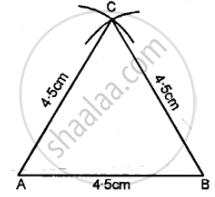# Construct Traingle Abc, When : Ab = Bc = Ca = 4.5 Cm. Measure All the Angles of the Triangle. Are They Equal ? - Mathematics

Diagram
Sum

Construct traingle ABC, when : AB = BC = CA = 4.5 cm. Measure all the angles of the triangle. Are they equal?

#### Solution

Steps of Construction:

(1) Draw a line AB = 4.5 cm.(2) Using compasses and taking BC as centre, draw an arc of 4.5 cm radius.

(3) With AC as centre, draw an arc of 4-5 cm radius, which cuts the previous arc at C.

(4) Join AC and BC.

(5) Measurement, ∠A = ∠B = ∠C = 60°.

Concept: Classification of Triangles (On the Basis of Sides, and of Angles)
Is there an error in this question or solution?

#### APPEARS IN

Selina Class 6 Mathematics
Chapter 26 Triangles (Including Types, Properties and Constructions)
Exercise 26 (B) | Q 4